# Divergent Integral

## divergent integral

[də′vər·jənt ′in·tə·grəl]
(mathematics)
An improper integral which does not have a finite value.

## Divergent Integral

an integral that has infinite limits of integration or an unbounded integrand and is either infinite or lacks a definite finite value. For example, the integral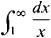defined as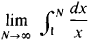diverges, since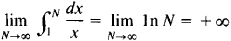On the other hand, the integral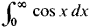diverges because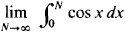does not exist.

References in periodicals archive ?
The technique of neglecting appropriately defined infinite quantities was devised by Hadamard, and the resulting finite value extracted from a divergent integral is referred to as the Hadamard finite part.
Application of this method with the method of regularization of divergent integrals  allowed to solve a number of problems for the lamellar shells with defects, such as [3 .
Paycha seeks to clarify some aspects of regularization techniques, which are used to make sense of divergent integrals, discrete sums, or traces.

Site: Follow: Share:
Open / Close